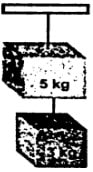Find the resultant of the following forces:

• 10 N along the x-axis
• 6 N along the y-axis and
• 4 N along the negative x-axis. (8.5 N making 45with x-axis)
Difficulty: Medium

Solution:

$F_{x}$ = Net force along x-axis = 10.4 = 6 N
$F_{y}$ = Force along y-axis = 5 N
The magnitude of the resultant force = F =?

F = $√F_{x}^{2} + F_{y}^{2}$
F = $√(6)^{2} + (6)^{2}$
F = √36 + 36
= √72 = 8.5 N

Now, θ = $tan^{-1}$ =$\frac{F_{y}}{F_{x}}$

θ = $tan^{-1}$ =$\frac{6}{6}$
θ = $tan^{-1}$ (1)
θ = 45⁰ with x-axis

Find the perpendicular components of a force of 50 N making an angle of 30⁰ with an x-axis. (43.3 N, 25 N)

Difficulty: Easy

Solution:

Force F = 50 N
Angle θ = 30⁰
$F_{x}$ = ? and $F_{y}$ = ?
$F_{x}$ = F cos θ
$F_{x}$= $50 \timescos 30$

= $50 N\times 0.866$ (˙.˙ cos 30⁰ = 0.866)
$F_{x}$ = 43.3 N

Similarly, $F_{y}$ = F sin θ
$F_{y}$= $50 \times 0.5$(˙.˙ sin 30⁰ = 0.5)
$F_{y}$= 25 N

Find the magnitude and direction of a force, if its x-component is 12 N and y-component is 5 N. (13 N making 22.6⁰ with x-axis)

Difficulty: Medium

Solution:

$F_{x}$ = 12 N

$F_{y}$= 5 N

1. Magnitude of the force = F = ?
2. Direction of the force = θ = ?

F = $√F_{x}^{2} + F_{y}^{2}$

F = $√(12)^{2} + (5)^{2}$

F = √144+25

F = 13 N

θ = $tan^{-1} =\frac{F_{y}}{F_{x}}$

θ = $tan^{-1} =\frac{12}{5}$

θ = $tan^{-1}$ (2.4)

θ = 22.6⁰ with x-axis

A force of 100 N is applied perpendicularly on a spanner at a distance of 10 cm from a nut. Find the torque produced by the force.  (10 Nm)

Difficulty: Easy

Solution:

Force = F = 100 N
Distance = L = 10 cm = 0.1 m
Torque Τ =?

Torque Τ = $F \times L$
= 100 $N \times 0.1 m$
= 10 Nm

A force is acting on a body making an angle of 30⁰ with the horizontal. The horizontal component of the force is 20 N. Find the force.  (23.1 N)

Difficulty: Easy

Solution:

Angle θ = 30⁰ (with x-axis)
Horizontal component of force $F_{x}$ = 20 N
Force F = ?
$F_{x}$ = F cos θ

20 N = F cos 30⁰
20 N = $F \times 0.866$ (˙.˙ cos 30⁰ = 0.866)
F =$\frac{ 20 N}{0.866}$= 23.09
F = 23.1 N

The steering of a car has a radius of 16 cm. Find the torque produced by a couple of 50 N. (16 Nm)

Difficulty: Easy

Solution:

Radius = r = L = 16 cm =$\frac{16}{100}$ m = 0.16 m
Couple arm = L = 16 cm =$\frac{16}{100 }$ m = 0.16 m
Force = F = 50 N
Torque Τ = ?

Torque Τ = $F \times L$
= $50 N\times(2 \times 0.16)$
= 16 Nm

A picture frame is hanging by two vertical strings. The tensions in the strings are 3.8 N and 4.4 N. Find the weight of the picture frame. (8.2 N)

Difficulty: Easy

Solution:

Tension $T_{1}$ = 3.8 N
Tension $T_{2}$ = 4.4 N
Weight of the picture frame = w =?

When the picture is in equilibrium, then

∑ $F_{x}$ = 0 and ∑ $F_{y}$ = 0
Therefore T – w = 0

Or $(T_{1} + T_{2})$ – w = 0
$T_{1} + T_{2}$ = w
3.8 + 4.4 = w
W = 8.2 N

Two blocks of mass 5 kg and 3 kg are suspended by the two strings as shown. Find the tension in each string. (80 N, 30 N)

Difficulty: Medium

Solution:

Mass of large block = M = 5 kg
Mass of large block = m = 3 kg
Tension produced in each string = $T_{1} = ? and T_{2}$ = ?

$T_{1}$ = $w_{1} + w_{2}$
$T_{1}$ = Mg + mg
$T_{1}$ = (M + m)g
$T_{1}$ = $(3+5) \times 10$
= $8 \times 10$
= 80 N

Also, $T_{2}$ = mg
$T_{2} = 3 \times10$ = 30 NA nut has been tightened by a force of 200 N using a 10 cm long spanner. What length of a spanner is required to loosen the same nut with 150 N force? (13.3 cm)

Difficulty: Medium

Solution:

Force = $F_{1}$ = 200 N
Length = $L_{1}$ = 10 cm = $\frac{10 }{100}$= 0.1 m

Length of the spanner to tighten the same nut:
Force = $F_{2}$ = 150 N
Length = $L_{2}$ = ?

Since $Τ_{1} = Τ_{2}$
$F_{1} \times L_{1}$ =  $F_{2} \times L_{2}$
$200 \times 0.1$ = $150 \times L_{2}$
20 = $150 \times L_{2}$
$L_{2}$ = $\frac{20}{150}$ = 0.133 m = $0.133 \times 100$ = 13.3 cm

A block of mass 10 kg is suspended at a distance of 20 cm from the center of a uniform bar 1 m long. What force is required to balance it at its center of gravity by applying the force at the other end of the bar?  (40 N)

Difficulty: Medium

Solution:

Mass of the block = m = 10 kg
Length of the bar = l = 1 m
Moment arm of $w_{1} = L_{1}$ = 20 cm = 0.2 m
Moment arm of $w_{2} = L_{2}$ = 50 cm = 0.5 m
Did force require to balance the bar $F_{2}$ =?

By applying principle of moments:

Clockwise moments = anticlockwise moments
$F_{1} \times L_{1}$ =  $F_{2} \times L_{2}$
$mg \times L_{1}$ =  $F_{2} \times L_{2}$
$(10 \times10 )\times 0.2$ =  $F_{2}\times0.5$
20 = $F_{2}\times 0.5$
$F_{2} =\frac{20}{0.5}$ = $\frac{200}{5}$= 40 N